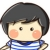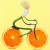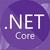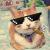# 日志分析想要一个正则

[DEBUG][20190513 00:00:00,300][org.apache.ibatis.logging.commons.JakartaCommonsLoggingImpl:49][] 任意文本内容

$1 DEBUG$2  20190513 00:00:00

$3 x.x.x.x$4 49

$5 任意文本内容 参考示例 # Default Tomcat catalina: # 30-Oct-2017 10:04:44.671 INFO [main] org.apache.catalina.startup.VersionLoggerListener.log Server version: Apache Tomcat/8.5.15 [COMMON] REGULAR=([\d\-a-zA-Z]+)\s([^ ]+)\s([A-Z]+)\s$([^$]+)\]\s([^ ]+)\s(.+) #Date Time Format, now only support these formats: # yyyy-MM-dd HH🇲🇲ss.SSS # yyyy-MM-dd HH🇲🇲ss # HH🇲🇲ss.SSS # HH🇲🇲ss TIME_FORMAT=HH🇲🇲ss.SSS [REGMAP] ITEM_TIME=$2
ITEM_LEVEL=$3 ITEM_PID= ITEM_TID=$4
ITEM_MODULE=$5 ITEM_FUN= ITEM_LOG=$6

### 以下是问题补充：

@骑猪飙高速：@沙枣 看图 https://snag.gy/mfERHZ.jpg (2019/05/15 18:16)

0# Version: 20190521
#           日志分析想要一个正则 - OSCHINA
#           https://www.oschina.net/question/251973_2306245
# Author: Crifan

import re

gInputLogStrList = [
"[DEBUG][20190513 00:00:00,300][org.apache.ibatis.logging.commons.JakartaCommonsLoggingImpl:49][] 任意文本内容",
# "[INFO][30-Oct-2017 10:04:44.671][org.apache.catalina.startup.VersionLoggerListener.log Server:51][] Apache Tomcat/8.5.15",
"[INFO][20190521 16:15:21,528][org.apache.catalina.startup.VersionLoggerListener.log Server:51][] Apache Tomcat/8.5.15",
]

def extractLog():
for eachLogStr in gInputLogStrList:
print("eachLogStr=%s" % eachLogStr)

# foundLog = re.search("$(?P[A-Z]+)$", eachLogStr)
# foundLog = re.search("$(?P[A-Z]+)$\s*$(?P[\w\s\-\.\,\:]+)$", eachLogStr)
# foundLog = re.search("$(?P[A-Z]+)$\s*$(?P[^\[$]+)\]", eachLogStr)
# foundLog = re.search("$(?P[A-Z]+)$$(?P[^\[$]+)\]$(?P[^:]+):(?P\d+)$", eachLogStr)

# Named Group = 带 命名组
# foundLog = re.search("^$(?P[A-Z]+)$$(?P[^\[$]+)\]$(?P[^:]+):(?P\d+)$\s*(?P.+)$", eachLogStr) # 后记：如果确保时间格式是固定的：20190513 00:00:00,300，那么可以改为： foundLog = re.search("^$(?P[A-Z]+)$$(?P\d+\s+\d+:\d+:\d+),\d+$$(?P[^:]+):(?P\d+)$\s*(?P.+)$", eachLogStr)
if foundLog:
logLevel = foundLog.group("logLevel")
logTime = foundLog.group("logTime")
logModule = foundLog.group("logModule")
logLineNum = foundLog.group("logLineNum")
logContent = foundLog.group("logContent")
print("logLevel=%s, logTime=%s, logModule=%s, logLineNum=%s, logContent=%s" % (logLevel, logTime, logModule, logLineNum, logContent))

# No Named Group = 不带命名组
foundLog = re.search("^$([A-Z]+)$$(\d+\s+\d+:\d+:\d+),\d+$$([^:]+):(\d+)$\s*(.+)$", eachLogStr) if foundLog: logLevel = foundLog.group(1) logTime = foundLog.group(2) logModule = foundLog.group(3) logLineNum = foundLog.group(4) logContent = foundLog.group(5) print("logLevel=%s, logTime=%s, logModule=%s, logLineNum=%s, logContent=%s" % (logLevel, logTime, logModule, logLineNum, logContent)) # Final Result: # logLevel=DEBUG, logTime=20190513 00:00:00,300, logModule=org.apache.ibatis.logging.commons.JakartaCommonsLoggingImpl, logLineNum=49, logContent=任意文本内容 # logLevel=INFO, logTime=30-Oct-2017 10:04:44.671, logModule=org.apache.catalina.startup.VersionLoggerListener.log Server, logLineNum=51, logContent=Apache Tomcat/8.5.15 # logLevel=DEBUG, logTime=20190513 00:00:00, logModule=org.apache.ibatis.logging.commons.JakartaCommonsLoggingImpl, logLineNum=49, logContent=任意文本内容 # logLevel=INFO, logTime=20190521 16:15:21, logModule=org.apache.catalina.startup.VersionLoggerListener.log Server, logLineNum=51, logContent=Apache Tomcat/8.5.15 if __name__ == "__main__": extractLog()  说明： • 根据你贴出的“参考示例”，代码中的logLineNum，估计对应的是ITEM_TID，但是你此处明显只是 行号  • 是你自己搞错了，还是说明有误，需要你自己查证   • 代码中 包含2套规则，你根据自己情况选择使用  • 一个是命名的组 • 一个是 不带命名的组   • 如果想要把上述python的正则换成其他语言的正则，比如perl，php，java等，适当修改即可得到，我就不赘述了回复 @IT小香猪 : 已更新。用 (\d+\s+\d+:\d+:\d+),\d+ 匹配： 20190513 00:00:00 但忽略： ,300^$([A-Z]+)$$([^\[$]+)\]$([^:]+):(\d+)$\s*(.+)$ 正确的把我需要的数据分段了 ， 唯一有点不足的就是时间 那个地方 20190513 00:00:00,300 要是在精确到 20190513 00:00:00 就非常完美
0/$([^$]+)\]+/

/
$# 左括号 ( # 捕获结束 [^$]+ # 不是右括号的所有字符
)   # 捕获结束
\]  # 右括号
/

0[DEBUG][20190513 00:00:00,300][org.apache.ibatis.logging.commons.JakartaCommonsLoggingImpl:49][]

$DEBUG$$\d{8}\s*\d{2}\:\d{2}\:\d{2},\d{3}$$[\w\.]+\:\d{1,5}$$[\w]*$

1.中间以点间隔的是jar包名，这里没有考虑jar名只能以_或字母开首的规定。

2.jar包后则是0-65536，我直接以0-99999为区间，这里需要你一点点的来写，用正则来写数学范围很麻烦。

3.日志内容没有考虑中文，只有a-z数字_这些ascii字符。00